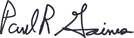Cart

# Expressing Sensitivity for ICP Applications

Zhongke asked Dr. Gaines about expressing sensitivity when working with ICP. Specifically, he wanted to know what is the unit of sensitivity and how sensitivity is calculated.

Dear Zhongke,

In analytical chemistry, sensitivity is the slope of the calibration curve. For ICP applications, it is expressed in counts/concentration units. When analysts are thinking about sensitivity, they use a language that expresses sensitivity in terms of detection limits (DL) rather than sensitivity units (S = counts /conc. units). DL is used because S has little value in making relative comparisons. It is much more to the point to speak in terms of DL rather than S. However, there is a relationship between S and DL. The relationship between the two is dependent upon the definition of DL. A popular definition of DL for ICP that incorporates sensitivity (S) is:

DL = (3(std. dev. of noise in counts)) / S

Using the above equation, the DL is expressed in the concentration units of choice. Using ICP as an example, a common concentration unit is ppm = micrograms of analyte/gram solution. Therefore, an analyst will state that a technique that has a DL of 0.001 ppm is 100 times more sensitive than a technique with a DL of 0.1 ppm. On a practical level, we think of sensitivity in terms of DL, even though we need to measure S to calculate the DL.